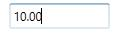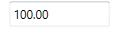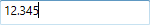# Restriction or Validation in WPF Double TextBox

This section explains how to validate or restrict the `DoubleTextBox` control value.

## Restrict the value within minimum and maximum value

The Value of the DoubleTextBox can be restricted within the maximum and minimum limits. Once the value has reached the maximum or minimum value , the value does not exceed the limit. We can change the maximum and minimum limits by using the MinValue property and MaxValue property.

You can choose when to validate the maximum and minimum limits while changing the values by using the MinValidation and MaxValidation properties.

• `OnKeyPress` — When setting the `MaxValidation` or `MinValidation` to `OnKeyPress`, the value in the `DoubleTextBox` will be validated shortly after pressing a key. So, it is not possible to provide any invalid input at all and the value does not exceed the maximum and minimum limits.

• `OnLostFocus` - When setting `MaxValidation` or `MinValidation` to `OnLostFocus`, the value in the `DoubleTextBox` is validated, when the `DoubleTextBox` loses the focus. That is, the `DoubleTextBox` will accept any value, validation will only take place after the `DoubleTextBox` has lost its keyboard focus. After validation, when the value of the `DoubleTextBox` is greater than the `MaxValue` or less than the `MinValue`, the value will be automatically set to `MaxValue` or `MinValue`.

• MaxValueOnExceedMaxDigit - When you give input greater than specified maximum limit, `MaxValueOnExceedMaxDigit` property will decide either it should retain the old value or reset to maximum limit that is specified. For example, if `MaxValue` is set to 100 and you are trying to input 200. `Value` will changed to 100 when `MaxValueOnExceedMaxDigit` is `true`. When `MaxValueOnExceedMaxDigit` is `false`, 20 will be retained and last entered 0 will be ignored.

NOTE

`MaxValueOnExceedMinDigit` property will be enabled only when the `MaxValidation` is set to `OnKeyPress`.

• MinValueOnExceedMinDigit - When you give input less than specified minimum limit, `MinValueOnExceedMinDigit` property will decide either it should retain the old value or reset to minimum limit that is specified. For example, if `MinValue` is set to 200 and the `Value` is 205 and you are trying change the value to 20. `Value` will changed to 200 when `MinValueOnExceedMinDigit` is `true`. When `MinValueOnExceedMinDigit` is `false`, Old value 205 will be retained.

NOTE

`MinValueOnExceedMinDigit` will be enabled only when the `MinValidation` is set to `OnKeyPress`.

``````<syncfusion:DoubleTextBox x:Name="doubleTextBox" Width="150" MaxValue="100" MinValue="10"
MinValueOnExceedMinDigit="True" MaxValueOnExceedMaxDigit="True"
MinValidation="OnKeyPress" MaxValidation="OnLostFocus"/>``````
``````DoubleTextBox doubleTextBox = new DoubleTextBox();
doubleTextBox.Width = 150;
doubleTextBox.Height = 25;
doubleTextBox.MinValue = 10;
doubleTextBox.MaxValue =100;
doubleTextBox.MinValidation = MinValidation.OnKeyPress;
doubleTextBox.MaxValidation = MaxValidation.OnLostFocus;
doubleTextBox.MinValueOnExceedMinDigit = true;
doubleTextBox.MaxValueOnExceedMaxDigit = true;``````

`MinValidation` is set to OnKeyPress, it cannot let to enter a value less than the `MinValue`. If try to enter a value less than the `MinValue`, then the `MinValue` will set to the `Value` property because `MinValueOnExceedMinDigit` is set to `true`.`MaxValidation` is set to OnLostFocus, so the `MaxValidation` will be performed only in the lost focus.## Restrict number of decimal digit

The `DoubleTextbox` allows the user to customize the number of digits to be displayed after the decimal point of `DoubleTextBox` value
by using NumberDecimalDigits property.
The decimal digit can be restricted within the maximum and minimum limits. Once the decimal digit has reached the maximum or minimum digit, the decimal digits does not exceed the limit. We can change the maximum and minimum decimal digits limit by using the MinimumNumberDecimalDigits and MaximumNumberDecimalDigits

``````<syncfusion:DoubleTextBox x:Name="doubleTextBox" Height="25" Width="150"
NumberDecimalDigits = "3" MinimumNumberDecimalDigits = "2"
MaximumNumberDecimalDigits = "5"/>``````
``````DoubleTextBox doubleTextBox = new DoubleTextBox();
doubleTextBox.Width = 150;
doubleTextBox.Height = 25;
doubleTextBox.MinValue = 0;
doubleTextBox.MaxValue = 100;
doubleTextBox.NumberDecimalDigits = 3;
doubleTextBox.MinimumNumberDecimalDigits = 2;
doubleTextBox.MaximumNumberDecimalDigits = 5;``````NOTE

`NumberDecimalDigits` property value will be allows set based on the in-between range of `MinimumNumberDecimalDigits` and `MaximumNumberDecimalDigits` property values.

The `DoubleTextBox` cannot allow the user input, edits when IsReadOnly property is sets to `true`. The user can still select text and display the cursor on the `DoubleTextBox` by setting the IsReadOnlyCaretVisible property to `true`. However, value can be changed programmatically in readonly mode.
``<syncfusion:DoubleTextBox x:Name="doubleTextBox" IsReadOnly="True" Value="10" IsReadOnlyCaretVisible="True"/>``
``````DoubleTextBox doubleTextBox = new DoubleTextBox();